# Texas Go Math Grade 3 Lesson 11.2 Answer Key Division Rules for 1 and 0

Refer to our Texas Go Math Grade 3 Answer Key Pdf to score good marks in the exams. Test yourself by practicing the problems from Texas Go Math Grade 3 Lesson 11.2 Answer Key Division Rules for 1 and 0.

## Texas Go Math Grade 3 Lesson 11.2 Answer Key Division Rules for 1 and 0

Essential Question
What are the rules for dividing with 1 and 0?
A Number Divided by 1 a1=a Just like multiplying by 1,
dividing any number by 1 doesn’t change the number at all.
0 Divided by a Number 0a=0 Dividing 0 by any number gives us a zero.
Zero will never change when multiplying or dividing any number by it.

Unlock the Problem

What rules for division can help you divide with 1 and 0?
Any number divided by 0 is not defined.
Any number (except 0) divided by itself equals 1.
Any number divided by 1 equals that number.
Explanation:
For Example:
35 ÷ 1 = 35
35 ÷ 0 = 0

If there is only 1 fishbowl, then all the fish must go in that fishbowl.Try This! There are 3 fish and 1 fishbowl. Draw a quick picture to show the fish in the fishbowl.

Write the equation your picture shows.3 ÷ 1 = 3

Rule A: Any number divided by 1 equals that number.

Math Talk
Mathematical processes
Explain how Rule A is related to the Identity Property of Multiplication.

If there is the same number of fish and fishbowls, then 1 fish goes in each fishbowl.Try This There are 3 fish and 3 fishbowls. Draw a quick picture to show the fish divided equally among the fishbowls.

Write the equation your picture shows.
3 ÷3 = 1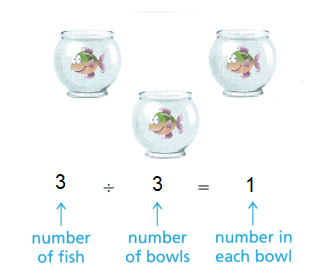Rule B: Any number (except 0) divided by itself equals 1.

If there are 0 fish and 4 fishbowls, there will not be any fish in the fishbowls.Try This! There are 0 fish and 3 fishbowls. Draw a quick picture to show the fishbowls.

Write the equation your picture shows.
___ ÷ ___ = ____
0 ÷ 4 = 0
Rule C: Zero divided by any number (except 0) equals 0.

If there are 0 fish bowls, then you cannot separate the fish equally into fishbowls Dividing by 0 is not possible.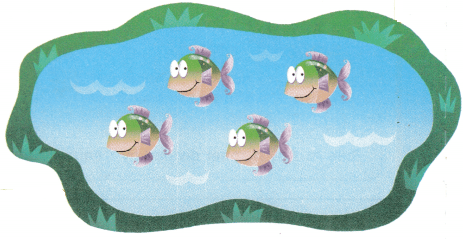Rule D: You cannot divide by 0.

Share and Show

Math Talk
Mathematical Processes
Explain what happens when you divide a number (except 0) by itself.

Question 1.
Use the picture to find 2 ÷ 2. ___Explanation:
Rule B: Any number (except 0) divided by itself equals 1.
2 ÷ 2 = 1

Find the quotient.

Question 2.
7 ÷ 1 = ___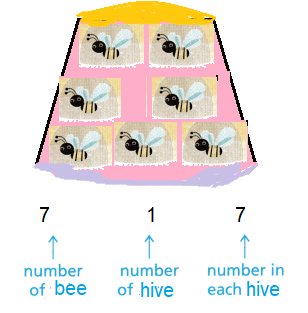Rule A: Any number divided by 1 equals that number.
7 ÷ 1 = 7

Question 3.
8 ÷ 8 = ___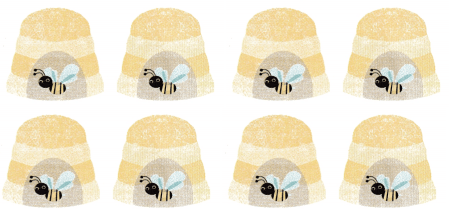8 ÷ 8 = 1
Explanation:
Rule A: Any number divided by 1 equals that number.

Question 4.
0 ÷ 5 = ___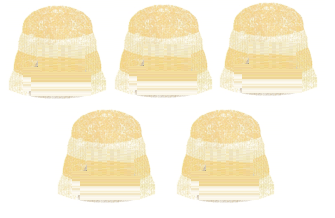0 ÷ 5 = 0
Explanation:
Rule C: Zero divided by any number (except 0) equals 0.

Question 5.
6 ÷ 6 = ___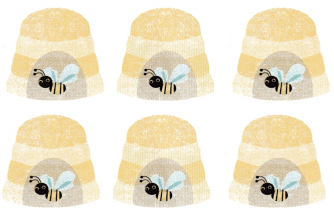Explanation:
Rule A: Any number divided by 1 equals that number.

Question 6.0 ÷ 5 = 0
Explanation:
Rule C: Zero divided by any number (except 0) equals 0.

Question 7.9 ÷ 1 = 9
Explanation:
Rule A: Any number divided by 1 equals that number

Question 8.7 ÷ 7 = 1
Explanation:
Rule A: Any number divided by 1 equals that number.

Question 9.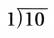10 ÷ 1 =10
Explanation:
Rule A: Any number divided by 1 equals that number

Problem Solving

Question 10.
Multi-Step Claire has 7 parakeets. She puts 4 of them in a cage. She divides the other parakeets equally among 3 friends to hold. How many parakeets does each friend get to hold?Explanation:
Claire has 7 parakeets.
She puts 4 of them in a cage.
7 – 4 = 3
She divides the other parakeets equally among 3 friends to hold.
Number of parakeets each friend get to hold
3 ÷ 3 = 1

Question 11.
Write Math Pose a Problem Look back at Problem 10. Change the number of parakeets and friends so you can use the equation 6 ÷ 6 = 1. Then solve your problem.
Claire has 10 parakeets. She puts 4 of them in a cage. She divides the other parakeets equally among 6 friends to hold. How many parakeets does each friend get to hold?
Explanation:
Claire has 10 parakeets.
She puts 4 of them in a cage.
10 – 4 = 6
She divides the other parakeets equally among 6 friends to hold.
Number of parakeets each friend get to hold
6 ÷ 6 = 1

Question 12.
Lily has some parrots. She gives each parrot 1 grape. If Lily gives out 5 grapes, how many parrots does she have?
Explanation:
Lily has some parrots.
She gives each parrot 1 grape.
If Lily gives out 5 grapes,
Total parrots she have
5 ÷ 5 = 1

Question 13.
H.O.T. Use Math Language Suppose a pet store has 21 birds that are in 21 cages. Use what you know about division rules to find the number of birds in each cage. Explain your answer.
21 ÷ 21 = 1
Any number divides by itself equals to 1
Explanation:
A pet store has 21 birds that are in 21 cages.
Division equation to find the number of birds in each cage.
21 ÷ 21 = 1

Fill in the bubble for the correct answer choice.

Question 14.
There are 18 people waiting in line to ride the Texas Giant roller coaster. There are 0 seats available and 6 roller coaster cars. Which division equation describes how many people can move from the line to a car?
(A) 0 × 6 = 0
(B) 6 ÷ 6 = 1
(C) 0 ÷ 6 = 0
(D) 6 ÷ 1 = 6
Option(C)
Explanation:
There are 18 people waiting in line to ride the Texas Giant roller coaster.
There are 0 seats available and 6 roller coaster cars.
Division equation 0 ÷ 6 = 0 describes number of people can move from the line to a car

Question 15.
Kai has some cats. Each cat has its own food bowl. There are 4 food bowls. How many cats does Kai have?
(A) 1
(B) 4
(C) 8
(D) 0
Option(B)
Explanation:
There are 4 food bowls.
Each cat has its own food bowl.
Number of cats Kai have 4 ÷ 1 = 4

Question 16.
Analyze Multi-Step Taylor has 6 juice boxes in her refrigerator. She leaves 2 juice boxes in the refrigerator and divides the rest equally among 3 friends and herself. How many juice boxes is each person given?
(A) 4
(B) 2
(C) 1
(D) 3
Option(C)
Explanation:
Taylor has 6 juice boxes in her refrigerator.
She leaves 2 juice boxes in the refrigerator
6 – 2 = 4
and divides the rest equally among 3 friends and herself.
Number of juice boxes each person get
4 ÷ 4 = 1

Texas Test Prep

Question 17.
Jude has 6 horses. Two horses are in the pasture, and he puts each of the rest of the horses in its own stall. How many stalls does Jude use?
(A) 2
(B) 1
(C) 0
(D) 4
Option(D)
Explanation:
Jude has 6 horses.
Two horses are in the pasture,
6 – 2 = 4
and he puts each of the rest of the horses in its own stall.
Number of stalls Jude use
4 ÷ 1 = 4

### Texas Go Math Grade 3 Lesson 11.2 Homework and Practice Answer Key

Question 1.
Use the picture to find 3 ÷ 1. ___3 ÷ 1 = 3
Explanation:
Rule A: Any number divided by 1 equals that number.

Question 2.
Use the picture to find 3 ÷ 3.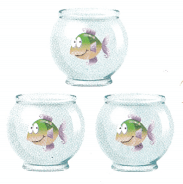3 ÷ 3 = 1
Explanation;
Rule B: Any number (except 0) divided by itself equals 1.

Find the quotient.

Question 3.
8 ÷ 1 = ___
8  ÷ 1 = 8
Explanation:
Rule A: Any number divided by 1 equals that number.

Question 4.
5 ÷ 5 = ___
5 ÷ 5 = 1
Explanation:
Rule B: Any number (except 0) divided by itself equals 1.

Question 5.
0 ÷ 9 = ___
0 ÷ 9 = 0
Explanation:
Rule C: Zero divided by any number (except 0) equals 0.

Question 6.
2 ÷ 2 = ___
Explanation:
Rule B: Any number (except 0) divided by itself equals 1.

Question 7.
0 ÷ 6 = ___
Explanation:
Rule C: Zero divided by any number (except 0) equals 0.

Question 8.
4 ÷ 1 = ___
Explanation:
Rule A: Any number divided by 1 equals that number.

Problem Solving

Question 9.
Angie has 8 muffins. She wraps up 4 muffins. She divides the other muffins among 3 friends and herself. How many muffins does each person get?
Each person get 1 muffin.
Explanation:
Angie has 8 muffins.
She wraps up 4 muffins.
8 – 4 = 4
She divides the other muffins among 3 friends and herself.
Number of muffins each person get
Rule B: Any number (except 0) divided by itself equals 1.
4 ÷ 4 = 1

Question 10.
Walt has 5 vases. He has no flowers to put in the vases. Write a division sentence to show how many flowers he can put in each vase.
0 ÷ 5 = 0
Explanation:
Walt has 5 vases.
He has no flowers to put in the vases.
Division equation 0 ÷ 5 = 0 show number of flowers he can put in each vase.

Question 11.
Look back at Problem 10. Change the number of flowers and vases so you can use the equation 7 ÷ 7 = 1.
Walt has 7 vases. He has 7 flowers to put in the vases. Write a division sentence to show how many flowers he can put in each vase.
Explanation:
Walt has 7 vases.
He has 7 flowers to put in the vases.
Division equation 7 ÷ 7 = 1 number of flowers he can put in each vase.

Texas Test Prep

Lesson Checks

Question 12.
There are 10 people waiting for the bus. There are no seats left on the bus. Which division equation describes how many people can get on the bus?
(A) 10 ÷ 10 = 1
(B) 10 × o = o
(C) 10 ÷ 1 = 10
(D) o ÷ 10 = o
Option(D)
Explanation:
There are 10 people waiting for the bus.
There are no seats left on the bus.
Division equation 0 ÷ 10 = 0 describes number of people that can get on the bus.
As, we know any that divided by 0 is 0.

Question 13.
A group of 9 people are going on a trip. The van can seat 6 people. Which division equation shows how many people can get on the van?
(A) 9 × 1 = 9
(B) 6 × 1 = 6
(C) 9 + 9 = 1
(D) 6 ÷ 1 = 6
Option(D)
Explanation:
A group of 9 people are going on a trip.
The van can seat 6 people.
Division equation 6 ÷ 1 = 6 shows number of people that can get on the van.
As, the seating capacity of the van is 6.

Question 14.
There are some students on the playground. Each student has a seat on the swings. There are 6 swing seats. How many students are on the swings?
(A) 0
(B) 6
(C) 1
(D) 12
Option(B)
Explanation:
There are 6 swing seats.
Each student has a seat on the swing.
Number of students on the swings
6 ÷ 1 = 6

Question 15.
A day care center has some babies. Each baby has a bottle. There are 8 bottles. How many babies are in the day care center?
(A) 16
(B) 0
(C) 1
(D) 8
Option(D)
Explanation:
A day care center has some babies.
Each baby has a bottle.
There are 8 bottles.
Total babies in the day care center
8 ÷ 1 = 8

Question 16.
Multi-Step Len has a box of 8 granola bars. He leaves 5 bars in the box and divides the rest among 2 friends and himself. How many granola bars does each person get?
(A) 1
(B) 5
(C) 2
(D) 8
Option(A)
Explanation:
Len has a box of 8 granola bars.
He leaves 5 bars in the box
8 – 5 = 3
and divides the rest among 2 friends and himself.
Number of granola bars each person get
3 ÷ 3 = 1

Question 17.
Multi-Step Sonya has a pack of 12 crayons. She leaves 0 crayons in the box and divides the rest equally among 11 students and herself. How many crayons is each student given?
(A) 0
(B) 1
(C) 12
(D) 11